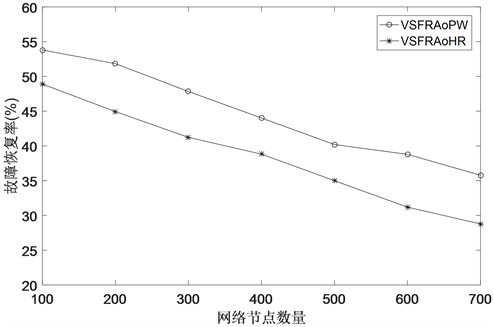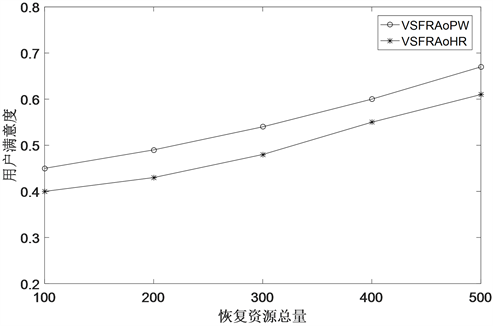﻿ 网络切片下基于粒子群的虚拟业务故障恢复算法

网络切片下基于粒子群的虚拟业务故障恢复算法Virtual Service Failure Recovery Algorithm Based on Particle Swarm in Network Slicing

Abstract: In a network slicing environment, how to restore as many virtual network services as possible under the constraints of limited resources is an urgent problem to be solved. To solve this problem, this paper models the virtual network service recovery problem as a problem of maximizing the number of failed services recovery. A particle swarm-based virtual service failure recovery algorithm under network slicing is proposed. The algorithm first constructs the faulty resource and virtual service as a two-layer correlation model. Secondly, the network resource recovery problem is modeled as a particle swarm problem, and the particle swarm optimization algorithm is used to solve it. In the experimental part, by comparing with existing algorithms, it is verified that the algorithm in this paper can recover more virtual network services that have failed.

1. 引言

2. 问题描述

3. 故障恢复模型

${\sum }_{m\in {F}^{n}}{R}_{m}^{n}r{e}_{m}^{n}\le R{e}^{n}$ (1)

${\sum }_{\left(mn\right)\in {F}^{e}}{R}_{mn}^{e}{r}_{mn}^{e}\le R{e}^{e}$ (2)

${\sum }_{{S}_{ij}^{v}\in {S}_{f}^{v}}{c}_{{S}_{ij}^{v}}^{m}{R}_{{S}_{ij}^{v}}\le {c}_{m}+\left({C}_{m}-{c}_{m}\right){R}_{m}^{n}-{C}_{g}$ (3)

${\sum }_{{S}_{ij}^{v}\in {S}_{f}^{v}}{e}_{{S}_{ij}^{v}}^{mn}{R}_{{S}_{ij}^{v}}\le {e}_{mn}+\left({B}_{mn}-{e}_{mn}\right){R}_{mn}^{e}-{B}_{g}$ (4)

$\mathrm{max}f\left({S}_{f}^{v}\right)={\sum }_{{S}_{ij}^{v}\in {S}_{f}^{v}}{R}_{{S}_{ij}^{v}}$ (5)

4. 算法

4.1. 算法分析

${V}_{i}\text{ }和\text{ }{V}_{j}\text{ }的优化策略={P}_{i}{V}_{i}\oplus {P}_{j}{V}_{j}$ (6)

${X}_{i}\text{ }和\text{ }{X}_{j}\text{ }区别={X}_{i}\Theta {X}_{j}$ (7)

$粒子位置\text{ }{X}_{i}\text{ }的新位置={X}_{i}\otimes {V}_{i}$ (8)

${X}_{i+1}={X}_{i}\otimes {V}_{i+1}$ (9)

${V}_{i+1}={P}_{1}{V}_{i}\oplus {P}_{2}\left({X}_{pb}\Theta {X}_{i}\right)\oplus {P}_{3}\left({X}_{gb}\Theta {X}_{i}\right)$ (10)

4.2. 算法描述Table 1. Virtual service failure recovery algorithm based on particle swarm

5. 性能分析

$u=\frac{\alpha {\sum }_{\theta \in \Theta }flo{w}_{\theta }+\beta {\sum }_{\theta \in \Theta }{f}_{\theta }}{\alpha {\sum }_{\varphi \in \Omega }flo{w}_{\varphi }+\beta {\sum }_{\varphi \in \Omega }{f}_{\varphi }}$ (11)Figure 1. Comparison of failure recovery rates of virtual businessFigure 2. The impact of total restoration resources on user satisfaction

6. 总结

 Peng, M., Li, Y., Jiang, J., et al. (2014) Heterogeneous Cloud Radio Access Networks: A New Perspective for Enhancing Spectral and Energy Efficiencies. IEEE Wireless Communications, 21, 126-135.
https://doi.org/10.1109/MWC.2014.7000980

 Tang, J., Tay, W.P. and Quek, T.Q.S. (2014) Cross-Layer Resource Allocation in Cloud Radio Access Network. 2014 IEEE Global Conference on Signal and Information Processing (GlobalSIP), Atlanta, 3-5 December 2014, 158-162.
https://doi.org/10.1109/GlobalSIP.2014.7032098

 Hawilo, H., Shami, A., Mirahmadi, M., et al. (2014) NFV: State of the Art, Challenges, and Implementation in Next Generation Mobile Networks (vEPC). IEEE Network, 28, 18-26.
https://doi.org/10.1109/MNET.2014.6963800

 Wang, X. (2011) Network Recovery and Augmentation under Geographically Correlated Region Failures. Proceedings of the Global Communications Conference, GLOBECOM 2011, Houston, 5-9 December 2011, 1-5.

 Mijumbi, R., Serrat, J., Gorricho, J.L., et al. (2015) Design and Evaluation of Algorithms for Mapping and Scheduling of Virtual Network Functions. Proceedings of the 2015 1st IEEE Conference on Network Softwarization (NetSoft), London, 13-17 April 2015, 1-9.
https://doi.org/10.1109/NETSOFT.2015.7116120

 Yu, H. and Yang, C. (2011) Partial Network Recovery to Maximize Traffic Demand. IEEE Communications Letters, 15, 1388-1390.
https://doi.org/10.1109/LCOMM.2011.103111.111668

 鲍宁海, 袁园, 刘自谦, 等. 基于链路生命期的光数据中心网络业务恢复方案[J]. 通信学报, 2018, 39(8): 125-132.

 潘志安, 刘庆杰, 王小英. 软件定义网络客户信息链路故障恢复仿真[J]. 计算机仿真, 2018(5): 241-244.

 Zegura, E.W., Calvert, K.L. and Bhattacharjee, S. (1996) How to Model an Internetwork. Proceedings of IEEE INFO- COM'96. Conference on Computer Communications, San Francisco, 24-28 March 1996, 594-602.

Top